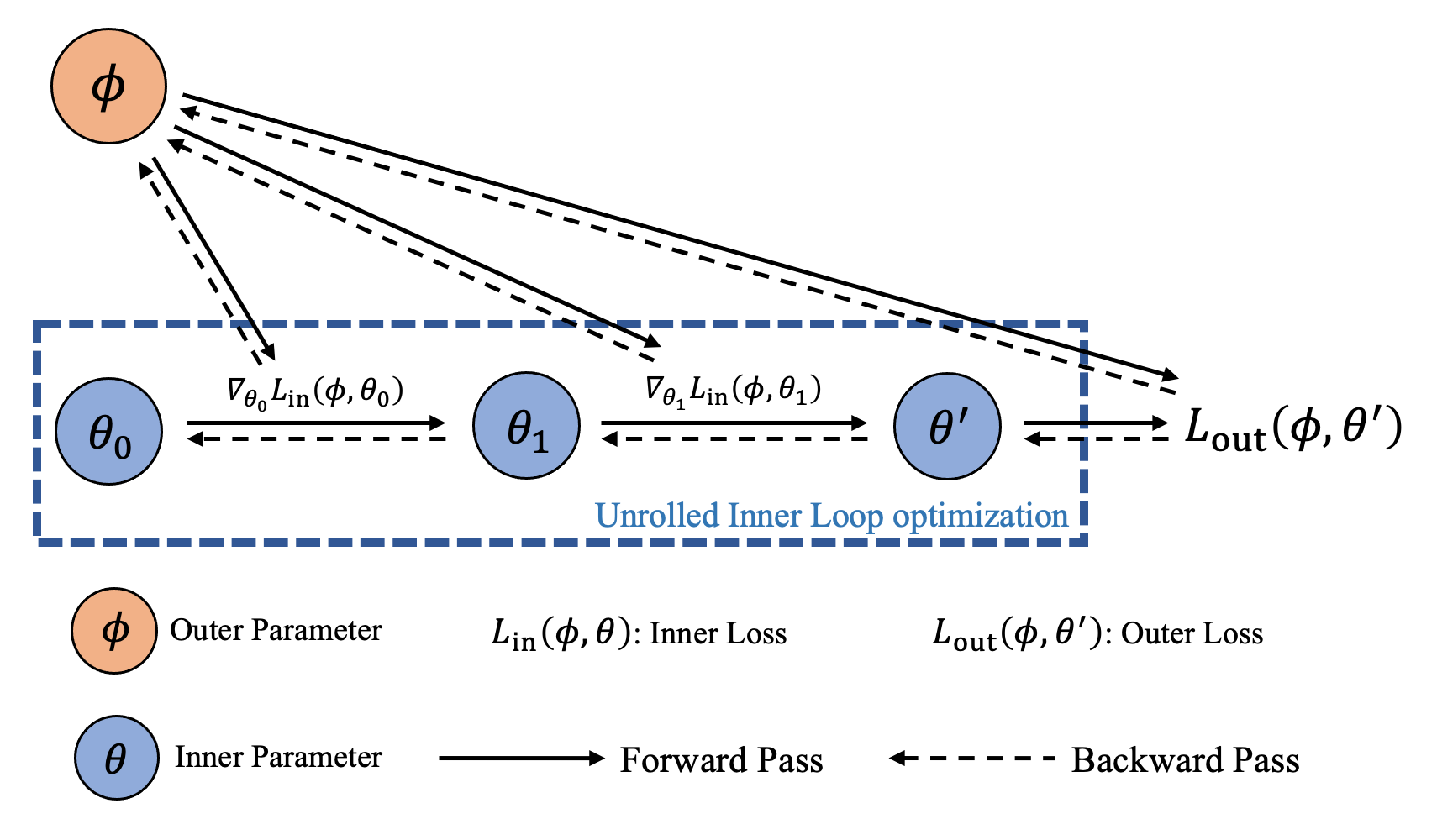The idea of explicit gradient is to treat the gradient step as a differentiable function and try to backpropagate through the unrolled optimization path. Namely, given

$\boldsymbol{\theta}^{\prime} (\boldsymbol{\phi}) \triangleq \boldsymbol{\theta}_0 - \alpha \sum_{i=0}^{K-1} \nabla_{\boldsymbol{\theta}_i} \mathcal{L}^{\text{in}} (\boldsymbol{\phi},\boldsymbol{\theta}_i),$

we would like to compute the gradient $$\nabla_{\boldsymbol{\phi}} \boldsymbol{\theta}^{\prime} (\boldsymbol{\phi})$$. This is usually done by AutoDiff through an inner optimization’s unrolled iterates.

## Differentiable Functional Optimizers

By passing the argument inplace as False to the update functions, we can make the optimization differentiable. Here is an example of making torchopt.adam() differentiable.

opt = torchopt.adam()
# Define meta and inner parameters
meta_params = ...
fmodel, params = make_functional(model)
# Initialize optimizer state
state = opt.init(params)

for iter in range(iter_times):
loss = inner_loss(fmodel, params, meta_params)
# Apply non-inplace parameter update

loss = outer_loss(fmodel, params, meta_params)


## Differentiable OOP Meta-Optimizers

For PyTorch-like API (e.g., step()), we designed a base class torchopt.MetaOptimizer to wrap our functional optimizers to become differentiable OOP meta-optimizers.

 torchopt.MetaOptimizer(module, impl) The base class for high-level differentiable optimizers. torchopt.MetaAdaDelta(module[, lr, rho, ...]) The differentiable AdaDelta optimizer. torchopt.MetaAdadelta alias of MetaAdaDelta torchopt.MetaAdaGrad(module[, lr, lr_decay, ...]) The differentiable AdaGrad optimizer. torchopt.MetaAdagrad alias of MetaAdaGrad torchopt.MetaAdam(module[, lr, betas, eps, ...]) The differentiable Adam optimizer. torchopt.MetaAdamW(module[, lr, betas, eps, ...]) The differentiable AdamW optimizer. torchopt.MetaAdaMax(module[, lr, betas, ...]) The differentiable AdaMax optimizer. torchopt.MetaAdamax alias of MetaAdaMax torchopt.MetaRAdam(module[, lr, betas, eps, ...]) The differentiable RAdam optimizer. torchopt.MetaRMSProp(module[, lr, alpha, ...]) The differentiable RMSProp optimizer. torchopt.MetaSGD(module, lr[, momentum, ...]) The differentiable Stochastic Gradient Descent optimizer.

By combining low-level API torchopt.MetaOptimizer with the previous functional optimizer, we can achieve high-level API:

# Low-level API
optim = torchopt.MetaOptimizer(net, torchopt.sgd(lr=1.0))

# High-level API
optim = torchopt.MetaSGD(net, lr=1.0)


Here is an example of using the OOP API torchopt.MetaAdam to conduct meta-gradient calculation.

# Define meta and inner parameters
meta_params = ...
model = ...
# Define differentiable optimizer

for iter in range(iter_times):
# Perform the inner update
loss = inner_loss(model, meta_params)
opt.step(loss)

loss = outer_loss(model, meta_params)
loss.backward()


### CPU/GPU Accelerated Optimizer

TorchOpt performs the symbolic reduction by manually writing the forward and backward functions using C++ OpenMP (CPU) and CUDA (GPU), which largely increase meta-gradient computational efficiency. Users can use accelerated optimizer by setting the use_accelerated_op as True. TorchOpt will automatically detect the device and allocate the corresponding accelerated optimizer.

# Check whether the accelerated_op is available:
torchopt.accelerated_op_available(torch.device('cpu'))

torchopt.accelerated_op_available(torch.device('cuda'))

net = Net(1).cuda()


## General Utilities

We provide the torchopt.extract_state_dict() and torchopt.recover_state_dict() functions to extract and restore the state of network and optimizer. By default, the extracted state dictionary is a reference (this design is for accumulating gradient of multi-task batch training, MAML for example). You can also set by='copy' to extract the copy of the state dictionary or set by='deepcopy' to have a detached copy.

 torchopt.extract_state_dict(target, *[, by, ...]) Extract target state. torchopt.recover_state_dict(target, state) Recover state. torchopt.stop_gradient(target) Stop the gradient for the input object.

Here is an usage example.

net = Net()

# Get the reference of state dictionary
init_net_state = torchopt.extract_state_dict(net, by='reference')
init_optim_state = torchopt.extract_state_dict(optim, by='reference')
# If set detach_buffers=True, the parameters are referenced as references while buffers are detached copies
init_net_state = torchopt.extract_state_dict(net, by='reference', detach_buffers=True)

# Set copy to get the copy of the state dictionary
init_net_state_copy = torchopt.extract_state_dict(net, by='copy')
init_optim_state_copy = torchopt.extract_state_dict(optim, by='copy')

# Set deepcopy to get the detached copy of state dictionary
init_net_state_deepcopy = torchopt.extract_state_dict(net, by='deepcopy')
init_optim_state_deepcopy = torchopt.extract_state_dict(optim, by='deepcopy')

# Conduct 2 inner-loop optimization
for i in range(2):
inner_loss = net(x)
optim.step(inner_loss)

print(f'a = {net.a!r}')

# Recover and reconduct 2 inner-loop optimization
torchopt.recover_state_dict(net, init_net_state)
torchopt.recover_state_dict(optim, init_optim_state)

for i in range(2):
inner_loss = net(x)
optim.step(inner_loss)

print(f'a = {net.a!r}')  # the same result


## Notebook Tutorial

Check the notebook tutorials at Meta Optimizer and Stop Gradient.